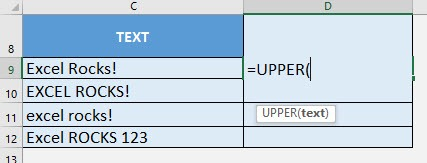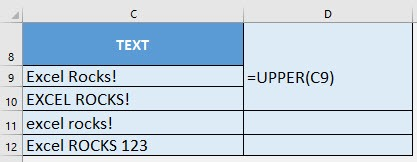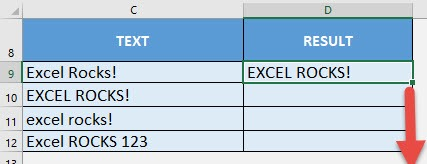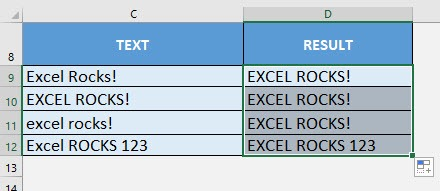What does it do?

Converts all characters in the text into uppercase

Formula breakdown:

=UPPER(text)

What it means:

=UPPER(text to be converted to upper case)

We can easily change text into upper case using the UPPER formula in Excel. It does not convert symbols and numbers though which we will see in the examples.

If you want to fix the uneven capitalization throughout the text, then this formula is perfect for you!

I explain how you can do this below:STEP 1: We need to enter the UPPER function in a blank cell:

## =UPPER(STEP 2: The UPPER arguments:

## text

What is the text to be converted to upper case?

Select the cell containing the text that you want to convert:

## =UPPER(C9)Apply the same formula to the rest of the cells by dragging the lower right corner downwards.You can see that the values are all now in upper case!How to Use the UPPER Formula in Excel